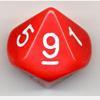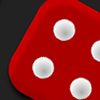Search by Topic

Filter by: Content type:
Age range:
Challenge level:

There are 9 NRICH Mathematical resources connected to Rounding, you may find related items under Numbers and the Number System.

Broad Topics > Numbers and the Number System > RoundingReasoned Rounding

Age 7 to 14 Challenge Level:

Four strategy dice games to consolidate pupils' understanding of rounding.Round the Dice Decimals 1

Age 7 to 11 Challenge Level:

Use two dice to generate two numbers with one decimal place. What happens when you round these numbers to the nearest whole number?Round the Two Dice

Age 5 to 7 Challenge Level:

This activity focuses on rounding to the nearest 10.Round the Dice Decimals 2

Age 7 to 11 Challenge Level:

What happens when you round these numbers to the nearest whole number?Round the Four Dice

Age 7 to 11 Challenge Level:

This activity involves rounding four-digit numbers to the nearest thousand.Rough Rectangle

Age 11 to 14 Short Challenge Level:

What is the smallest possible area that this rectangle could have?Round the Three Dice

Age 7 to 11 Challenge Level:

What happens when you round these three-digit numbers to the nearest 100?Archimedes and Numerical Roots

Age 14 to 16 Challenge Level:

The problem is how did Archimedes calculate the lengths of the sides of the polygons which needed him to be able to calculate square roots?Route to Root

Age 16 to 18 Challenge Level:

A sequence of numbers x1, x2, x3, ... starts with x1 = 2, and, if you know any term xn, you can find the next term xn+1 using the formula: xn+1 = (xn + 3/xn)/2 . Calculate the first six terms of this. . . .